### NUMERICAL METHODS IN ENGINEERING

Numerical Methods in Engineering (GTIAE)

Introductory course to Numerical Methods applied to engineering problems using Matlab. It corresponds to undergrad second year course at the ETSEIB (UPC-BarcelonaTech).

This material is only for educational purposes. You are free to download its contents.

Please, let me know if you find some typos or you have some ideas to improve it (toni.susin at upc.edu).

Mathworks award

Mathworks (the MATLAB's company) has rewarded the Numerical Factory project with an Academic Developement Support Grant. Congratulations to all team members!!!.

T0 - Numbers and Computers

Theory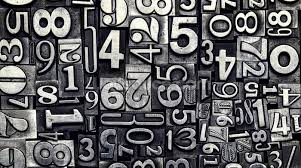Short introduction to numerical mathematics and computer number representation.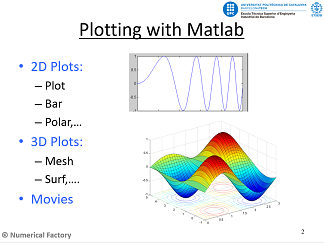How to plot using Matlab.How to manipulate images using Matlab.

Practices

P0.1 - Read and Write numbers using Matlab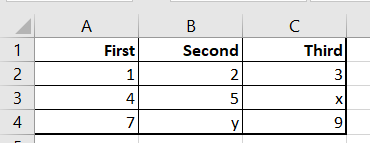Short introduction to managing numerical results using a txt file or a spreadsheet.

P0.2 - 2D-3D Plot Using MatlabShort introduction to the different possibilities of plotting data with Matlab.

P0.3 - Image Processing with Matlab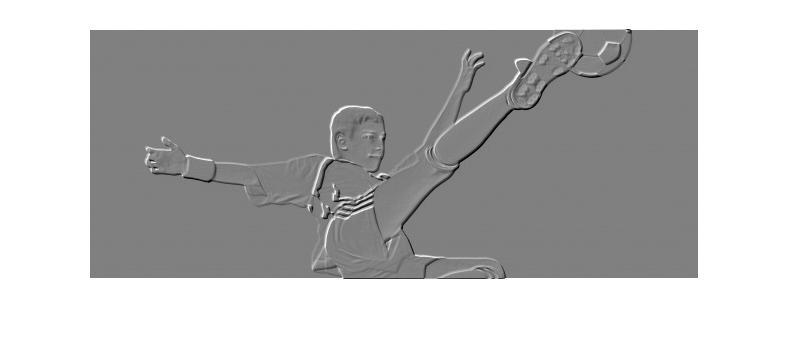Short introduction to image processing in Matlab.

T1 - Interpolation: Shape Functions

Theory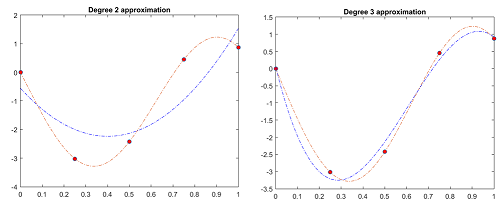In this presentation the following concepts are introduced: Interpolation. Lagrange Interpolation. Splines. Shape Functions 1D-2D. Triangular Elements. Quadrilateral Elements.

Practices

P1.1 - Interpolation 1D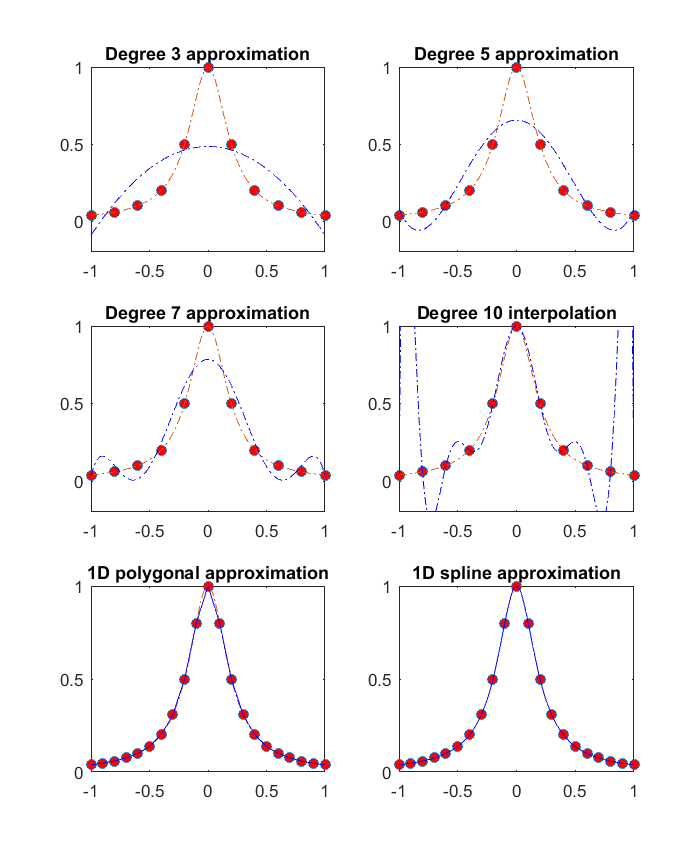Interpolation 1D . Introduction of the polyfit and polyval Matlab functions. Splines and interp1.

P1.2 - Triangle Shape Functions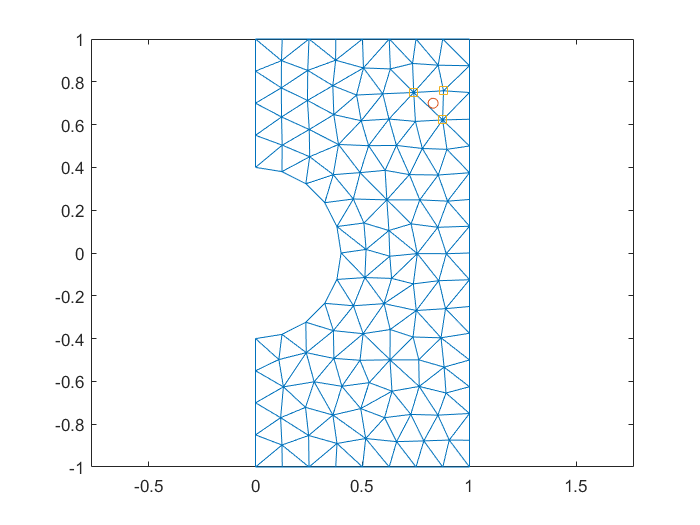Shape function for triangles . Load triangular mesh files. Barycentric coordinates. Is inside function.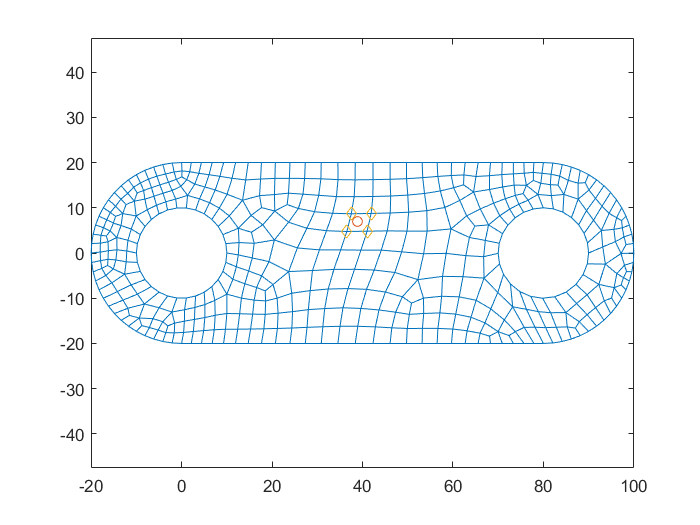Shape function for quadrilaterals . Barycentric coordinates for quadrilateral elements. Is inside function.

P1.4 - Color ResultsMesh results . Color interpolation. Processing images with Matlab.

P1.5 - Color Interpolation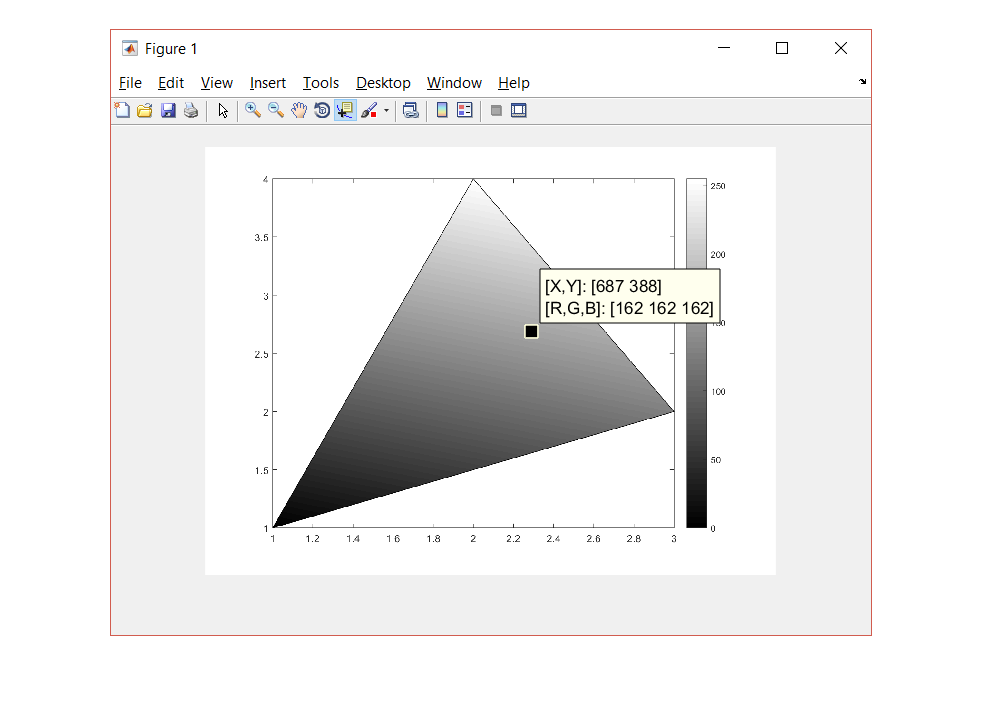How Matlab interpolates color in a triangle.

T2 - Iteration

Theory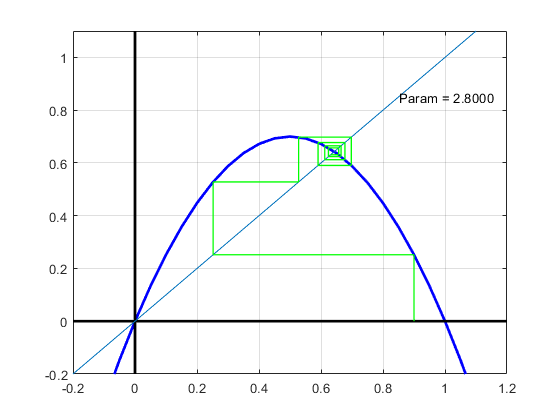In this presentation we introduce one of the most important tool in scientific computation, the iterative procedures. We will see different applications where iterative methods are the best approaches to the solution.

Practices

P2.1 - Iteration 1DIteration 1D . The concept of successive approach to the solution. Graphical Convergence and Chaotic systems.

P2.2 - Iteration 2D. Fractal sets. (students homework)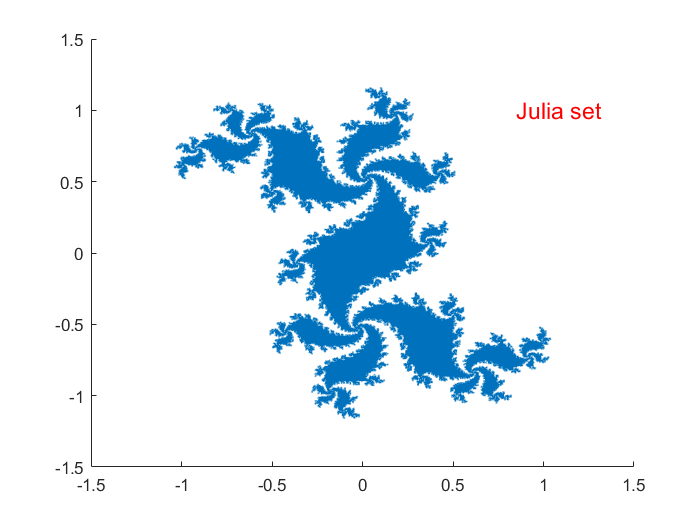Examples of Complex Iteration . Mandelbrot and Julia sets.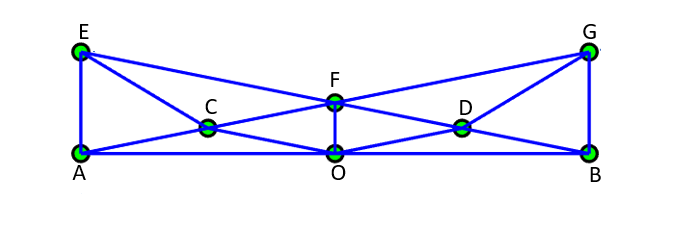Iterative Power method applied to the Google rank sort order for web pages.

T3 - Linear Systems

TheoryIn this presentation we introduce the different numerical methods to solve linear systems of equations from both direct and iterative approaches.

Practices

P3.1 - SVD: Image compressionSVD decomposition method applied to image compression.

P3.2 - SVD: Oriented Bounding BoxSVD decomposition method applied to compute the Bounding Box of a 3D model.

P3.3 - Capture Smartphone Sensor Data: Step Counter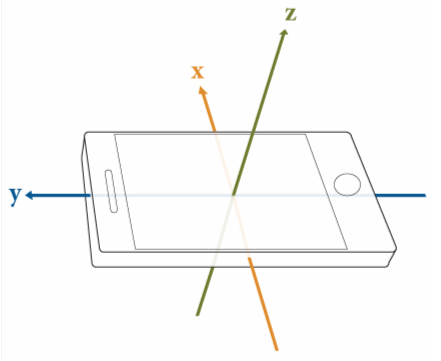Application to count the steps recorded from Matlab on your smartphone.

P3.4 - Iterative Methods for Linear Systems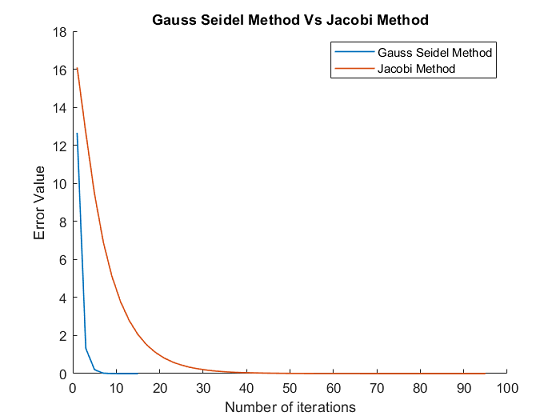Iterative methods applied to the solution of linear systems of equations. Jacobi, Gauss-Seidel and Gradient Conjugate Methods.

T4 - Partial Differential Equations (PDE)

TheoryIn this presentation we introduce the different numerical methods to approximate the solution of Partial Diffferential Equations (PDE) using Finite Differences.

Practices

P4.1 - Poisson's Equation: Sparse Matrix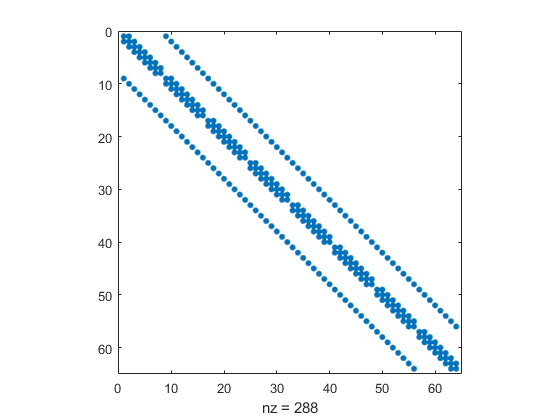Example of 2D Poisson's Partial Differential Equations using Finite Differences.

P4.2 - 1D Heat EquationExamples of 1D Partial Differential Equations using Finite Differences.

P4.3 - 2D Heat Equation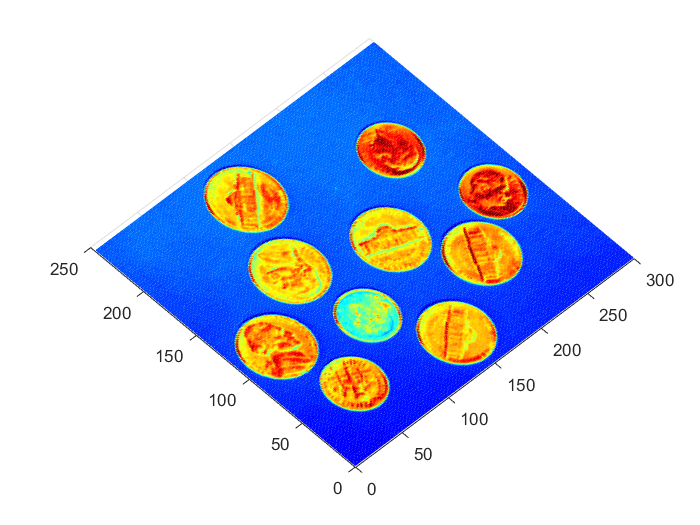Examples of 2D heat equation . Application to blur an image

P4.4 - 1D Wave Equation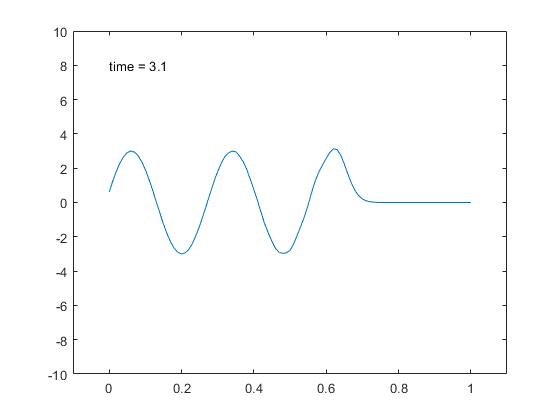Examples of 1D wave PDE using Finite Differences.

P4.5 - Wave Equation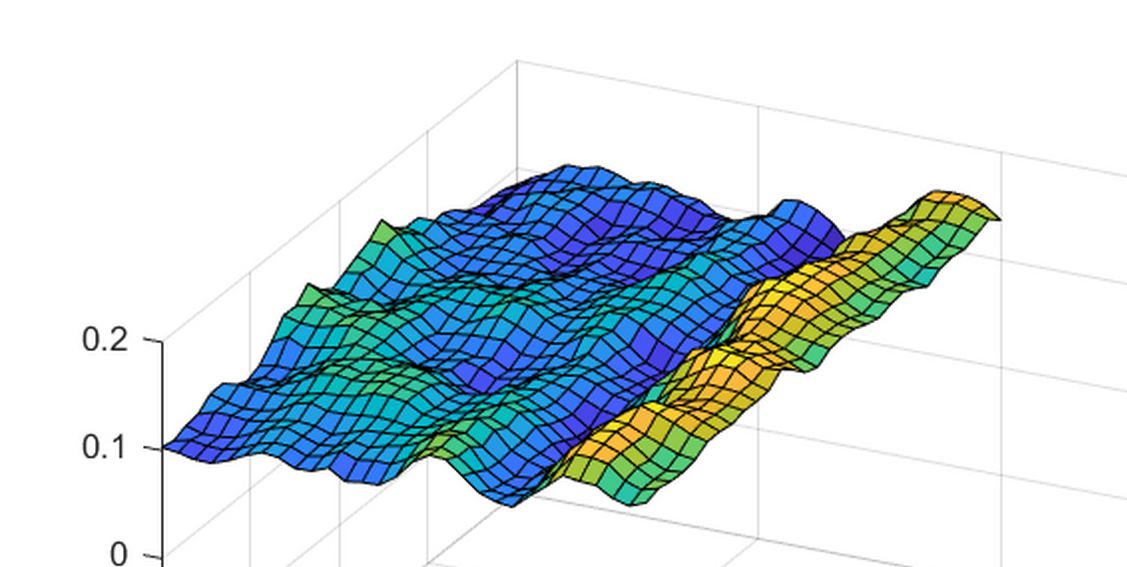Examples of Partial Differential Equations

T5 - Ordinary Differential Equations (ODE)

Theory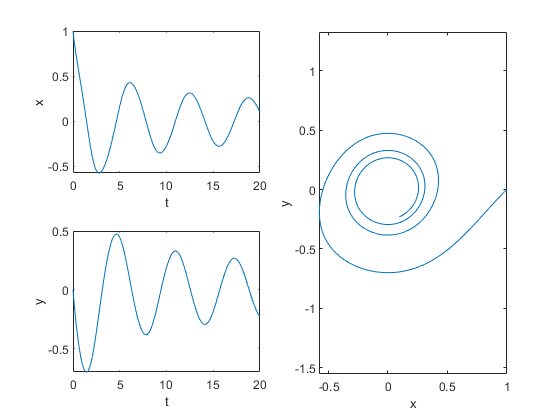In this presentation we introduce the different numerical methods to approximate the solution of Ordinary Diffferential Equations (ODE) using Initial Value Problems (IPV). Methods like Euler, Mid Point or Runge-Kutta are explained.

Practices

P5.1 - 1D ODE integration methods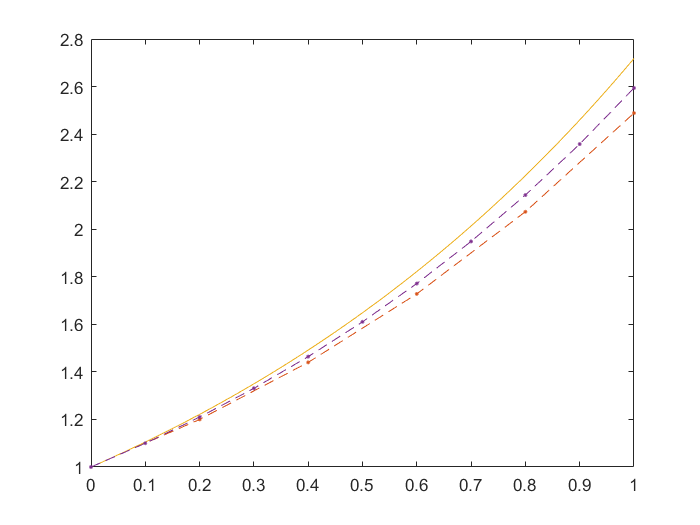Examples of 1D ordinary differential equations

P5.2 - 2D ODE integration methods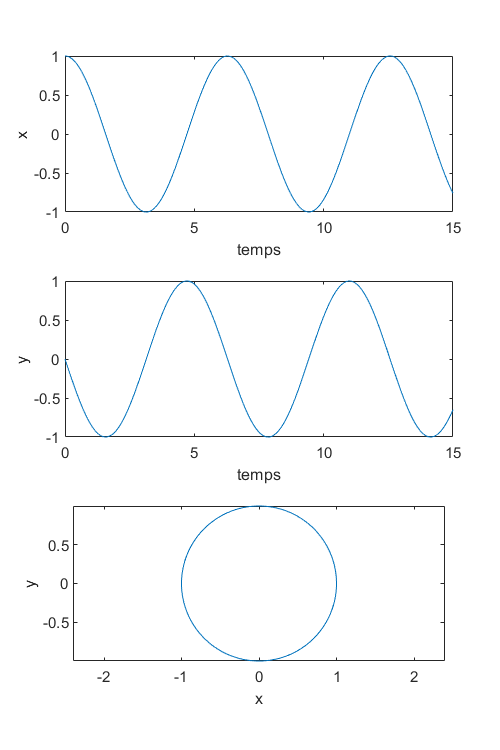Examples of 2D ordinary differential equations

Extra material Files

All mesh files needed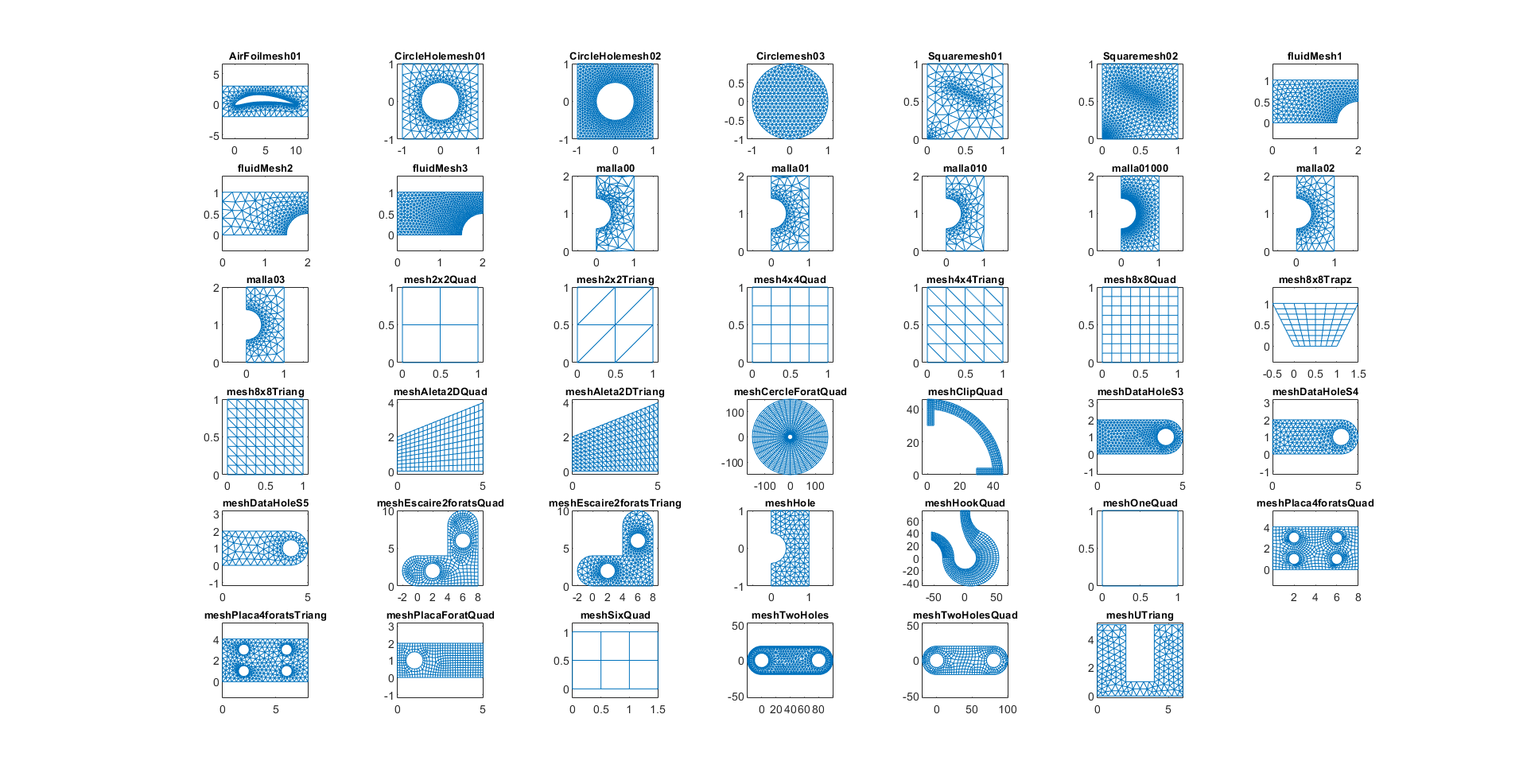Files containing the meshes used along the course.

Plot and extra files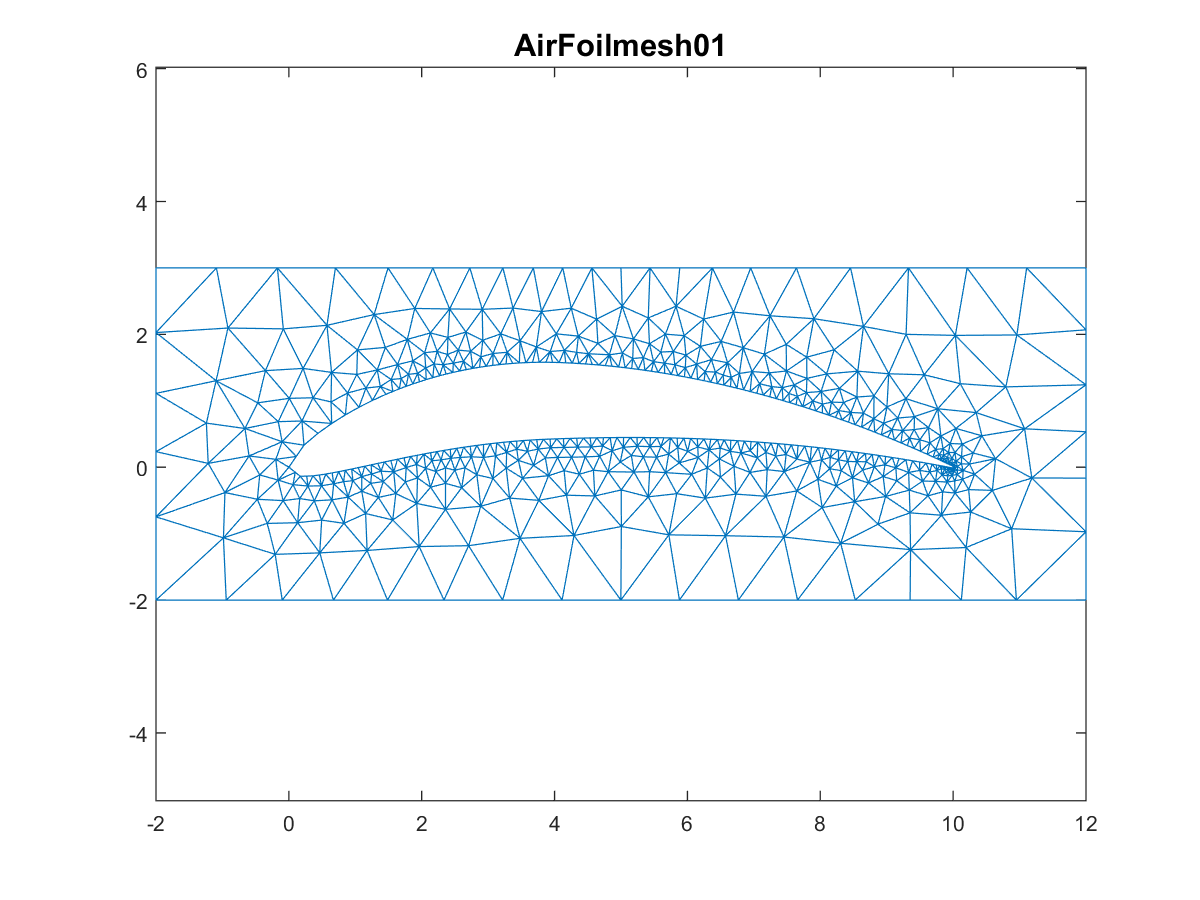Files containing all plot and some other extra files.